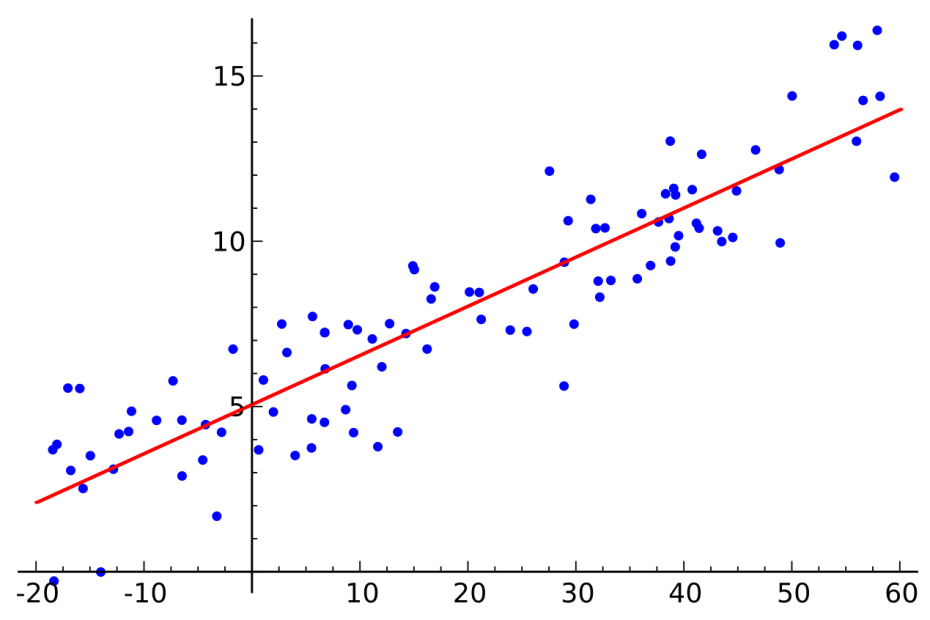# Regression Analysis## Simple Linear Regression

Simple Linear Regression is used to model the relationship between one predictor variable and one response variable. This is called ‘linear because the said relationship can be expressed in the form of the equation ‘y = mx + x”. And it is called ‘Simple’ because only one Predictor Variable is involved.